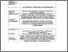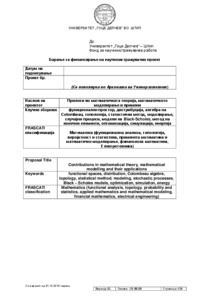# Contributions in mathematical theory, mathematical modelling and their applications

Atanasova-Pacemska, Tatjana and Jolevska-Tuneska, Biljana and Sarac, Vasilija and Lazarova, Limonka and Miteva, Marija and Karamazova, Elena and Timovski, Riste and Runcev, Kostadin and Srebrenova, Maja (2017) Contributions in mathematical theory, mathematical modelling and their applications. [Project]Preview Text Проект УГД - Прилози во мат.теорија, за е-репозиториум.pdf Download (843kB) | Preview

## Abstract

In the frame of this project we will give contributions in mathematical theory, as well as in mathematical modelling and its applications.
Within distribution theory, many operations with distributions, as well as product of distributions, composition of distributions and convolution product of distributions don’t always exist for arbitrary two distributions. Such operations are defined only for a class of ‘regular’ distributions. But, using some new approaches like neutrix calculus and Colombeau algebras the number of couples of distributions which product, convolution product and composition exist will be enlarged. The space of these distributions will also be considered from the topological point of view. The obtained results may be applied in solving many problems in physics, electrical engineering and energetics that can not be solved in the frame of classical distribution theory.
Considering topological spaces and their properties, in order to make generalizations, it is very important to determine the properties of such spaces that are transferred with continuous mapping. Such properties are called topological properties. Considering some processes and links between objects in topological spaces, the proofs of mathematical theorems and propositions is getting easier while considering topological properties. Thus, studying topological properties is always a challenge for mathematicians. In this context the research within this project will be done. The accent will be put on defining and studying the notion of ‘connectedness’ in topological spaces, components and quasicomponents in special topological spaces (for example uniform spaces and uniformly continuous mappings, proper mappings, raises, etc.) and different kind of connectedness, local connectedness, line local connectedness, so the contribution in mathematical theory and its application will be given.
Many of the obtained results will be relevant for application in economy, especially in studying financial markets. With this project we will try to obtain mathematical models of financial markets. We are planning to consider both discrete and continuous models (in time and state) of financial markets. The arbitrages as a way for realizing profit with minimal risk will also be considered.
Different methods and techniques for optimization will be explored and applied in solving appropriate problems.
In the area of developing and applying mathematical models for projection of power devices, mathematical models of electrical engines will be elaborated based on concrete specifications taken from the industrial producers, in a way to implement developed mathematical models in optimization software and in improvement of working characteristics of the engine referring to previously given target function for optimization. Obtained results will be relevant to the industry in terms of building prototypes of optimized versions of the machines, which after laboratory testing can be produces.

Item Type: Project Engineering and Technology > Electrical engineering, electronic engineering, information engineeringNatural sciences > Matematics Faculty of Electrical Engineering Tatjana A. Pacemska 08 Nov 2017 11:47 08 Nov 2017 11:47 https://eprints.ugd.edu.mk/id/eprint/18391View Item